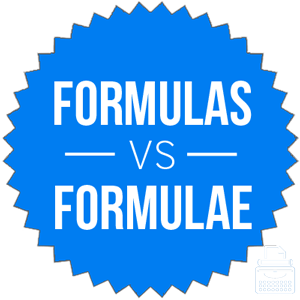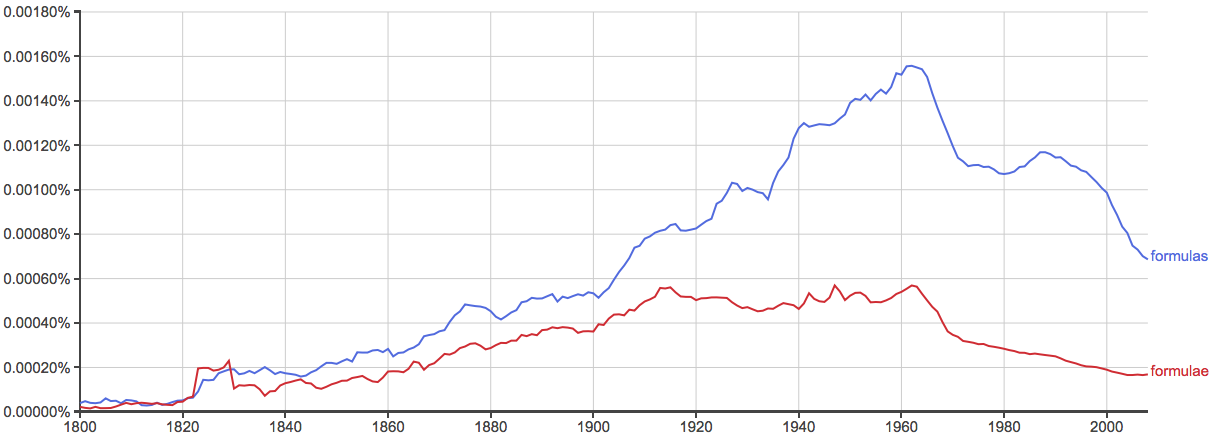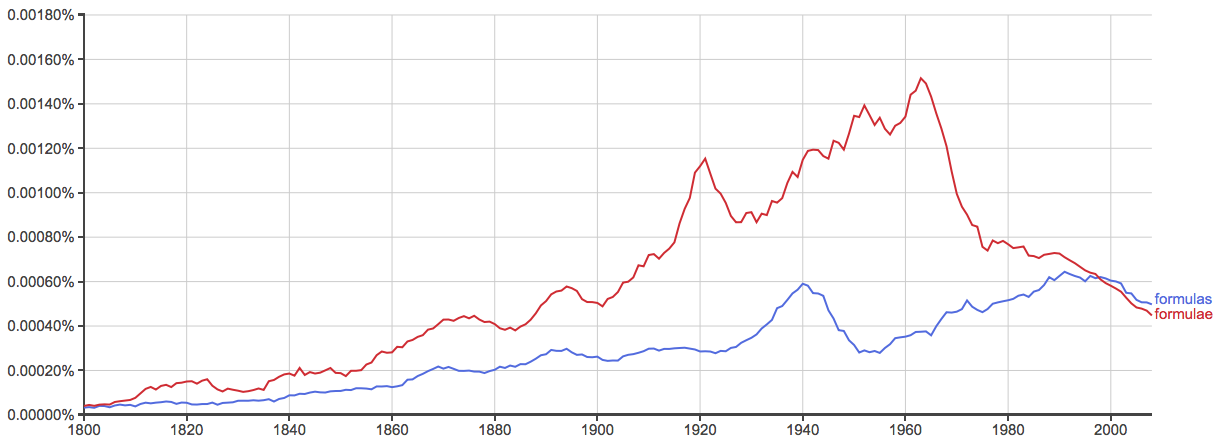# Formulas or Formulae – Which is Correct?

If you were an evil scientist working on a secret chemical that would allow you to control the minds of all humans, would you have a giant chalkboard full of formulae or formulas?

Given the subject material, formulae seems like a more natural choice for this context (at least to this writer). But in truth, formulas and formulae are both standard forms of this word in different language communities.

## What is the Difference Between Formulas and Formulae?

In this post, I will compare formulae vs. formulas. I will use each of these words in at least one example sentence, so you can see how they appear in context.

Plus, I will show you a useful memory tool that makes choosing formulae or formulas a little easier.

## When to Use FormulasWhat does formulas mean? Formulas is a plural of the word formula.

Formula is a noun that refers to either a specified mathematical computation or a list of ingredients used in various concoctions and potions.

Formula can also be a liquid fed to babies, because English is unpredictable sometimes.

For example,

• “You would’ve gotten the answer correct if you would have only followed my formulas!” cried the math teacher to his pupil.
• Gregory found a book of formulas in a neglected corner of the library, and discovered that it contained a recipe for love potion.

Formula comes from an old Latin word that means, very roughly translated, little form. It has been in use in English since the early 17th century.

## When to Use FormulaeWhat does formulae mean? Formulae is an alternative plural of formula. It means the same thing as formulas and is interchangeable in most contexts. According to Garner’s Modern English Usage, formulas predominates in all written uses with the exception of scientific writing.

In other words, if you are writing for a general circulation newspaper, you will probably want to use formulas. If you are writing in a scientific journal, you might consider using formulae.

Also, interestingly enough, formulae is more common in British English than American English (see below).

The -ae suffix in formulae is a Latin pluralization, so it’s likely closer to what the original Latin plural for this word would have been. Nonetheless, languages evolve over time to suit the needs of the people who use them, so formula adopted an Anglicized -s pluralization.

In the sentences above, formulae can substitute for formulas without changing the meaning of the sentences. They will seem slightly more pretentious to some American readers, though.

## Trick to Remember the Difference

You can use either formulas or formulae and achieve the same results. American audiences are used to seeing formulas, while British audiences will likely be familiar with both words, as you can see from the following charts.

Formulas vs. Formulae – American English:Formulas vs. Formulae – British English:These graphs aren’t scientific, since they only look at books published in English since 1800. Both formulas and formulae are older than 1800, but the charts give a clear picture of present-day usage trends.

Since formulas has the American -s pluralization, and there is an S in United States, it should be easy to remember that formulas is the American version of this plural noun.

## Summary

Is it formulas or formulae? Formulas and formulae are two plurals of the noun formula, which has a few different meanings. It can mean a mathematical computation or a recipe, depending on context.

• The plurals are interchangeable.
• Formulas is more common everywhere except scientific writings.

Contents